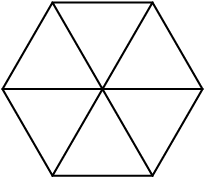5. Loops Practice Quiz and Assignment Work Period¶

Quick Overview of Day

Use for loops and functions to draw one shape elegantly. Continue working on a Python turtle graphics assignment, focused on repetition and conditionals.

5.1. Practice Problem¶Draw the shape above. You can choose to either:

• create a function, and repeatedly call that function
• use repetition without a function (if you choose this method, you will want to use a nested for loop)

5.2. What Does This Program Do?¶

Note

Your teacher may choose to use the following examples as a class activity, by displaying the examples, and having you take a guess as to what you think each will do before running the code.

What will the following programs output? Why?

5.3. Loops Practice Quiz¶

5.3.1. Question 1¶

loops-practice-quiz1: What shape will the turtle alex draw when the code below is executed?:

import turtle

the_window = turtle.Screen()
the_window.bgcolor("lightgreen")
alex = turtle.Turtle()
alex.pensize(3)

for i in [0,1,2,3]:
alex.forward(50)
alex.left(90)

• No shape will be drawn.
• Try again!
• A line segment.
• Great!
• A triangle.
• Try again!
• A square.
• Try again! Notice that that alex.left(90) command is not inside the for loop.

5.3.2. Question 2¶

loops-practice-quiz2: What shape will the turtle alex draw when the code below is executed?:

import turtle

the_window = turtle.Screen()
the_window.bgcolor("lightgreen")
alex = turtle.Turtle()
alex.pensize(3)

for i in [0,1,2,3]:
alex.forward(50)
alex.left(90)

• No shape will be drawn.
• Try again!
• A line segment.
• Try again! This time, the alex.left(90) is included in the for loop.
• A triangle.
• Try again!
• A square.
• Great!

5.3.3. Question 3¶

loops-practice-quiz3: In the following code, how many lines does this code print?:

for number in [10, 9, 8, 7, 6, 5, 4, 3, 2, 1, 0]:
print("I have", number, "cookies.  I'm going to eat one.")

5.3.4. Question 4¶

loops-practice-quiz4: The following will print a line showing the number 4:

for i in range(4):
print(i)

• True
• Nope. Remember that range(4) will create a list with elements [0,1,2,3].
• False
• Great!

5.3.5. Question 5¶

loops-practice-quiz5: What is the last line that this code will print?:

i = 1
while (i <= 3):
i = i + 1
print(i)

5.4. Turtle Graphics Assignment¶

Use the rest of this class time to keep working on your current Python assignment (possibly a turtle graphics drawing, with a focus on looping and conditionals).

Next Section - 1. Fruitful Functions and Variable Scope